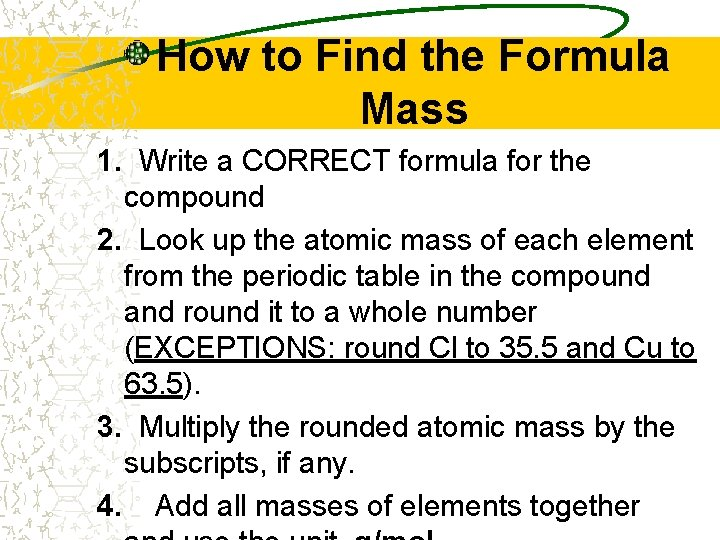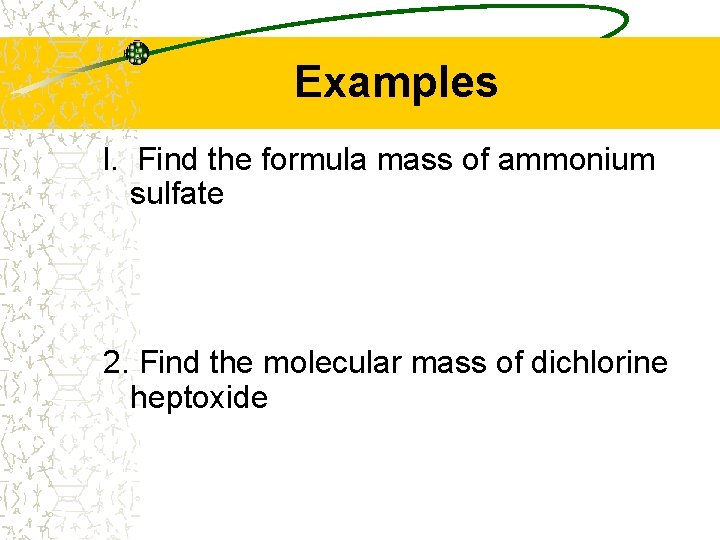# Formula Mass Formula mass the formula mass of

• Slides: 8Formula MassFormula mass – the formula mass of any molecule, formula unit, or ion is the sum of the average atomic masses of all the atoms represented in its formula. Molar mass- the molar mass of a substance is equal to the mass in grams of one mole, or 6. 022 x 1023 particles of the substance. NOTE- These are 2 different terms but both are calculated the same way. Know these terms, but usually we will refer to the molar mass as just that, or we may use “gfm” (theHow to Find the Formula Mass 1. Write a CORRECT formula for the compound 2. Look up the atomic mass of each element from the periodic table in the compound and round it to a whole number (EXCEPTIONS: round Cl to 35. 5 and Cu to 63. 5). 3. Multiply the rounded atomic mass by the subscripts, if any. 4. Add all masses of elements togetherExample Ex: Find the formula mass of calcium phosphate 1. Ca 3(PO 4)2 2. Ca = 40 x 3 = 120 3. P = 31 x 2 = 62 O = 16 x 8 = 128 310 g/molExamples l. Find the formula mass of ammonium sulfate 2. Find the molecular mass of dichlorine heptoxideHydrates Some compounds trap water inside their crystal structure and are known as hydrates. You will not be able to predict which compounds will form hydrates. All you have to do is to be able to name them and find their molar masses (including the water) Cu. SO 4 • 5 H 20 is an example of a hydrate. This says that one formula unitHydrates are named by naming the ionic compound by the regular rules and then adding (as a second word) a prefix indicating the number of water molecules. You will use the word “hydrate” to indicate water. The above compound would be called copper(II) sulfate pentahydrate. To find the formula mass of a hydrate, simply find the mass of the ionic compound by itself and then ADD thePractice 1. What is the formula mass of barium chloride dihydrate? 2. What is the formula mass of aluminum sulfate octahydrate?